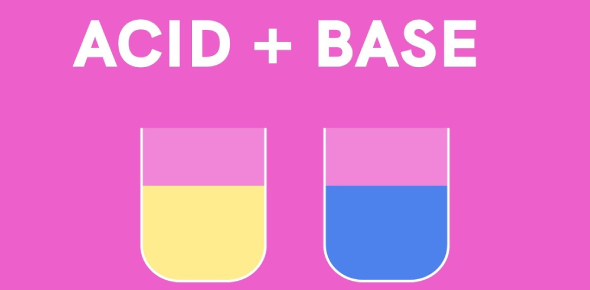# Acid And Bases Test: Titration Problems In Chemistry

8 Questions | Attempts: 11103
ShareSettingsTitration is a process of slowly adding one solution of a known concentration to a known volume of an unknown concentration until the reaction gets neutralized. This trivia quiz is based on the titration problem of acids and bases that we learned and had some practice in the lab this week. See how much you understood by taking this test!

• 1.
The Concentration of NaOH is 0.5 M if 20 ml is needed to titrate 35 mL of acid, what is the concentration of the acid?
• A.

0.875 M of acid

• B.

0.0029 M of the acid

• C.

0.29 M of the acid

• D.

0.00875 M of acid

• 2.
A 15.5 ml sample of 0.215 M KOH was titrated with a weak acid. It took 21.2 mL of the acid to reach the equivalence point. What is the molarity of the acid?
• A.

0.157 M acid

• B.

0.29 M acid

• C.

0.0157 M acid

• D.

0.029 M acid

• 3.
If 20.0 mL of 0.100 M HCl is titrated with 19.5 mL of a NaOH solution. What is the molarity of the NaOH solution?
• A.

0.0975 M NaOH

• B.

0.0103 M NaOH

• C.

0.103 M NaOH

• D.

0.975 M NaOH

• 4.
The Concentration of HCl, a strong acid is 0.5 M. If 20 ml HCl is needed to titrate 40 mL of NaOH, what is the Concentration of NaOH?
• A.

0.25 M NaOH

• B.

0.5 M NaOH

• C.

1 M NaOH

• D.

0.1 M NaOH

• 5.
The Concentration of LiOH is 0.50 M. If 25 mL of LiOH is needed to titrate 40 mL of HNO3 (nitric acid) what is the Concentration of HNO3?
• A.

0.03125 M HNO3

• B.

0.8 M HNO3

• C.

0.3125 M HNO3

• D.

0.08 M HNO3

• 6.
What is the purpose of an indicator in the solution with the unknown concentration?
• A.

It tells when there is enough acid in the solution.

• B.

It tells when the equivalence point is obtained.

• C.

It tells when there is enough base in the solution

• 7.
The Equation for the Neutralization reaction between solutions of Potassium hydroxide and Hydrochloric Acid is:
• A.

H+ (aq) + OH- (aq) --> H2O

• B.

KOH (aq) + HCl (aq) -->H2O (l) + KCl

• C.

POH (aq) + HClO (aq) --> H2O (l) + PClO (aq)

• D.

KOH (aq) + HCl (aq) -->K2Cl (aq)+ H2O (l)

• E.

KOH (aq) + HCl (aq) --> K2Cl (aq)+ H2O (l) + H+

• 8.
Calculate the molarity of a HCl solution if 25.0 mL of the solution is  neutralized by 15.5 mL of 0.800 M NaOH:
• A.

0.248 M

• B.

0.496 M

• C.

1.29 M

• D.

0.645 M

## Related TopicsBack to top
×

Wait!
Here's an interesting quiz for you.